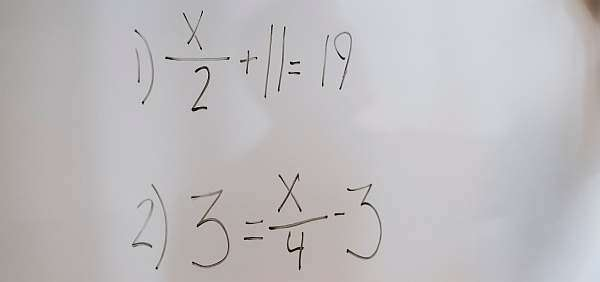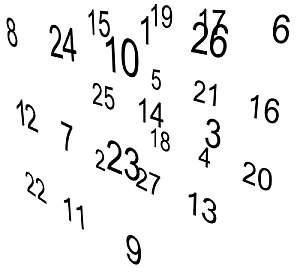# Solve for y Calculator

Instructions: You can use this calculator to get a step-by-step solution of how to solve for y from a provided linear equation. Please type a valid linear equation in the box provided below.Type a linear equation (Ex: 3x - 2y = 3, x - y= 2/3 x, etc.)

## More about solving for y from a linear equation

One of the most common algebraic processes is that of solving for one specific variable in terms of the other variables from an equation

In layman terms, that means using algebraic manipulation properties so that we "isolate" one variable on one side of the equation, and the rest of variables, if any, are kept on the other side.

We all know that solving equations could be tricky, because equations could be complex, and there may not be a clear path for manipulating things around so that to have one variable alone on one side of the equation.

This is not the case with linear equations, where the procedure is easier, almost straightforward. All you need to make sure is that the arithmetic operations are done correctly## How do you solve for Y?

It is the same as when you solve for any variable: you manipulate the terms so that you leave the term you want to solve for on one side and the rest of variables on the other side.

For example, if you solve the equation $$3x + y = 3$$, you need to have $$y$$ alone on the left side, so you subtract $$3x$$ to the whole equation, which has the effect of "passing $$3x$$ to the other side with negative sign".

This technique of "passing a term to the other side with a changed sign" is normalized as the de facto way of manipulating equations, as it would be a bit too tedious to be justifying every single step.

## How do you solve for Y on a calculator?

Not all calculators will have this feature. For our calculator, all you need to type is a valid linear equation, such as for example "2x - 3y = 3" (without the quotes).

All calculators that you could potentially use will ask you for a way to specify the equation.

## Solving for y with steps

So, how do you find the value of y in an equation?

In layman terms, you need to "isolate" y on one side of the equation and all the rest on the other side.

All you have to do to get all the steps of solving $$y$$ from a linear equation, you just have to type the equation in the box provided. Just make sure it is a valid linear equation.

Similarly you could use this solve for x calculator if you wanted to solve for x instead of y.Example of solving for y for a given linear equation

Solve for y in the given equation $$2x + 3y = 1$$.

We have been provided with the following equation:

$\displaystyle 2x+3y=\frac{3}{2}x+3y+3$

Passing all the variables and their coefficients to the left of the equation, and grouping the constants on the right, we get:

$\displaystyle \left(2-\frac{3}{2}\right)x+\left(3-3\right)y = 3$

and simplifying all the terms that need simplification, we get

$\displaystyle x=6$

and we observe that the terms with $$y$$ cancel out, so then what we have is a vertical line.

Conclusion: Based on the equation provided, we observe that the terms that include $$y$$ cancel out, and we conclude that we cannot solve for $$y$$.